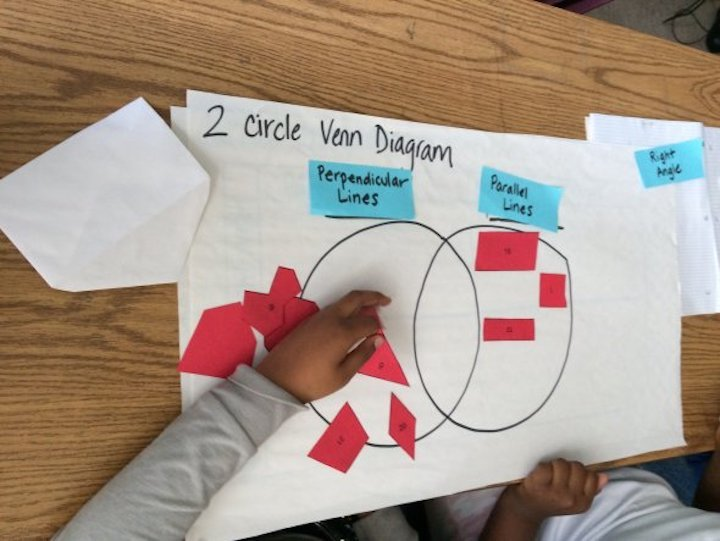## Overview### In Grade 4, instructional time should focus on three critical areas:

1. developing understanding and fluency with multi-digit multiplication and developing understanding of dividing to find quotients involving multi-digit dividends;
2. developing an understanding of fraction equivalence, addition, and subtraction of fractions with like denominators, and multiplication of fractions by whole numbers;
3. understanding that geometric figures can be analyzed and classified based on their properties, such as having parallel sides, perpendicular sides, particular angle measures, and symmetry.